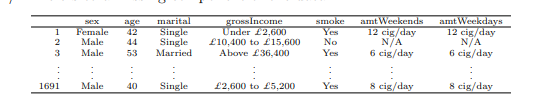## SolutionA UK resident.

1691.

### c) Indicate whether each variable in the study is numerical or categorical. If numerical, identify as continuous or discrete. If categorical, indicate if the variable is ordinal.

Sex is categorical.

Age is numerical (discrete).

Marital status is categorical.

Gross income is categorical (ordinal).

Whether a person smokes is categorical.

Amount of cigarettes per day on weekends is numerical (discrete).

Amount of cigarettes per day on weekdays is numerical (discrete).